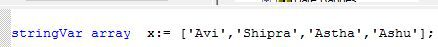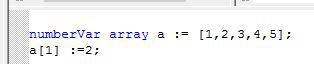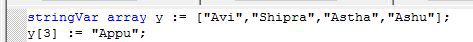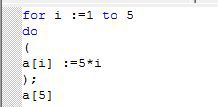# Creating Arrays

An array is the collection of the homogeneous data type. In Crystal Report, array variable can be defined as an array keyword. We can include two types of an array,
• Static
• DynamicWe can also assign fixed position to the elements of an array and this position is fixed in the formula.

For ExampleWe can also adjust the data between the list of an array. Assign any column name of the inserting data and apply the formula.

For ExampleArray with Loops

We can also use the loops in an array. With the loops, we can repeat the data or numbers many times according to the conditions. An example of an array with the loops are as follows,This will show the 25 output.

Summary

Thus, we learnt the use of an array in Crystal Report.
Next » Parameters